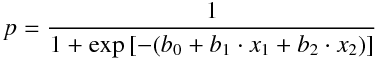Skip to content

# adopy/adopy

Adaptive Design Optimization for Experimental Tasks
Python MakefileJaeyeong Yang Update citation
Latest commit 0fb543e Jul 4, 2019
Type Name Latest commit message Commit time
Failed to load latest commit information.adopy Jul 2, 2019docs Jul 4, 2019examples Jul 2, 2019tests Jun 19, 2019.editorconfig Jan 17, 2019.gitignore Jun 16, 2019.travis.ymlLICENSE Oct 30, 2018MANIFEST.in Jan 8, 2019Makefile Jul 2, 2019Pipfile Jul 2, 2019README.md Jul 4, 2019codecov.yml Jun 12, 2019setup.cfgsetup.py Jul 2, 2019

# ADOpyADOpy is a Python implementation of Adaptive Design Optimization (ADO; Myung, Cavagnaro, & Pitt, 2013), which computes optimal designs dynamically in an experiment. Its modular structure permit easy integration into existing experimentation code.

ADOpy supports Python 3.5 or above and relies on NumPy, SciPy, and Pandas.

### Features

• Grid-based computation of optimal designs using only three classes: `adopy.Task`, `adopy.Model`, and `adopy.Engine`.
• Easily customizable for your own tasks and models
• Pre-implemented Task and Model classes including:
• Psychometric function estimation for 2AFC tasks (`adopy.tasks.psi`)
• Delay discounting task (`adopy.tasks.ddt`)
• Choice under risk and ambiguity task (`adopy.tasks.cra`)
• Example code for experiments using PsychoPy (link)

## (not so) Quick-start guide

### Step 0. Install ADOpy on the terminal

```# Install the stable version from PyPI
pip install adopy

# Or install the developmental version from GitHub
git clone https://github.com/adopy/adopy.git
cd adopy
git checkout develop
pip install .```

### Step 1. Define a task using `adopy.Task`

Assume that a user want to use ADOpy for an arbitrary task with two design variables (`x1` and `x2`) where participants can make a binary choice on each trial. Then, the task can be defined with `adopy.Task` as described below:

```from adopy import Task

task = Task(name='My New Experiment',  # Name of the task (optional)
designs = ['x1', 'x2'],    # Labels of design variables
responses = [0, 1])        # Possible responses```

### Step 2. Define a model using `adopy.Model`

To predict partipants' choices, here we assume a logistic regression model that calculates the probability to make a positive response using three model parameters (`b0`, `b1`, and `b2`):How to compute the probabilty `p` should be defined as a function:

```import numpy as np

def calculate_prob(x1, x2, b0, b1, b2):
"""A function to compute the probability of a positive response."""
logit = b0 + x1 * b1 + x1 * b2
p_obs = 1. / (1 + np.exp(-logit))
return p_obs```

Using the information and the function, the model can be defined with `adopy.Model`:

```from adopy import Model

model = Model(name='My Logistic Model',   # Name of the model (optional)
params=['b0', 'b1', 'b2'],  # Labels of model parameters
func=calculate_prob)        # A probability function```

### Step 3. Define grids for design variables and model parameters

Since ADOpy uses grid search to search the design space and parameter space, you must define a grid for design variables and model parameters. The grid can be defined using the labels (of design variables or model parameters) as its key and an array of the corresponding grid points as its value.

```import numpy as np

grid_design = {
'x1': np.linspace(0, 50, 100),    # 100 grid points within [0, 50]
'x2': np.linspace(-20, 30, 100),  # 100 grid points within [-20, 30]
}

grid_param = {
'b0': np.linspace(-5, 5, 100),  # 100 grid points within [-5, 5]
'b1': np.linspace(-5, 5, 100),  # 100 grid points within [-5, 5]
'b2': np.linspace(-5, 5, 100),  # 100 grid points within [-5, 5]
}```

### Step 4. Initialize an engine using `adopy.Engine`

Using the objects created so far, an engine should be initialized using `adopy.Engine`. It contains built-in functions to compute an optimal design using ADO.

```from adopy import Engine

engine = Engine(model=model,              # a Model object
task=task,                # a Task object
grid_design=grid_design,  # a grid for design variables
grid_param=grid_param)    # a grid for model parameters```

### Step 5. Compute a design using the engine

```# Compute an optimal design using ADO
design = engine.get_design()
design = engine.get_design('optimal')

# Compute a randomly chosen design, as is typically done in non-ADO experiments
design = engine.get_design('random')```

### Step 6. Collect an observation in your experiment

```# Get a response from a participant using your own code
response = ...```

### Step 7. Update the engine with the observation

```# Update the engine with the design and the corresponding response
engine.update(design, response)```

### Step 8. Repeat Step 5 through Step 7 until the experiment is over

```NUM_TRIAL = 100  # number of trials

for trial in range(NUM_TRIAL):
# Compute an optimal design for the current trial
design = engine.get_design('optimal')

# Get a response using the optimal design
response = ...  # Using users' own codes

# Update the engine
engine.update(design, response)```

## Citation

If you use ADOpy, please cite this package along with the specific version. It greatly encourages contributors to continue supporting ADOpy.

Yang, J., Pitt, M. A., Ahn, W., & Myung, J. I. (2019). ADOpy: A Python Package for Adaptive Design Optimization. https://doi.org/10.31234/osf.io/mdu23

## References

• Myung, J. I., Cavagnaro, D. R., and Pitt, M. A. (2013). A tutorial on adaptive design optimization. Journal of Mathematical Psychology, 57, 53–67.
You can’t perform that action at this time.